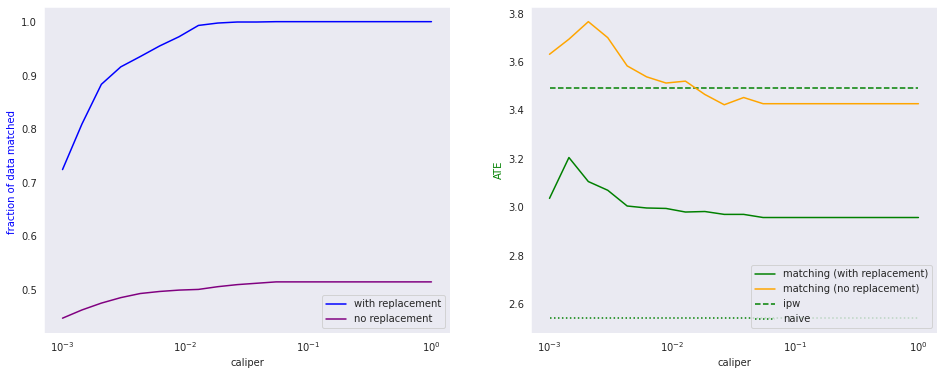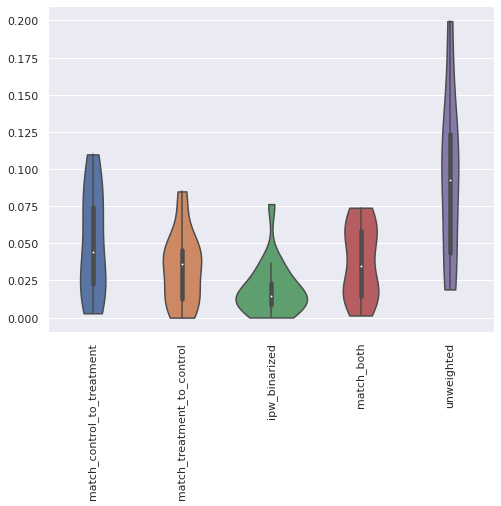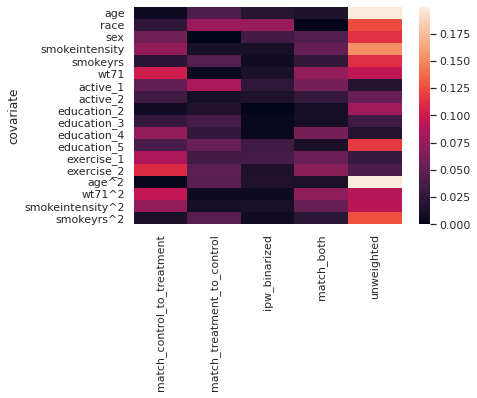# Matching Model¶

To find the expected effect of the intervention on the population, we match each treated individual with one or more untreated individuals which are "almost the same" as him or her.

In :
from causallib.estimation import IPW, Matching
import matplotlib.pyplot as plt
import seaborn as sb
import pandas as pd
import numpy as np
from causallib.evaluation.weight_evaluator import calculate_covariate_balance
from sklearn.linear_model import LogisticRegression
from causallib.preprocessing.transformers import PropensityTransformer, MatchingTransformer
from causallib.evaluation import PropensityEvaluator
%matplotlib inline


#### Data:¶

The effect of quitting to smoke on weight loss.
Data example is taken from Hernan and Robins Causal Inference Book

When we are looking for nearby data points to match against, the one hot encoding may not be the best choice. Augmented features are also not needed and may introduce bias (eg, if we inlude age^2, we will call one pair of subjects more distant in the age variable than another pair if the base age is older, even though the difference is the same ). So we do not augment the continuous variables, and instead of one hot encoding the categorical variables, we binarize them to "high/low" values.

In :
def binarize(df, column_name):
df = df.copy()
m = df[column_name].median()
def balance(i): return np.abs(0.5 - (df[column_name] < i).sum()/len(df))
mstar = min([m-1, m, m+1], key=balance)
df = df.assign(**{column_name: (df[column_name] < mstar).astype(int)})
df = df.rename(columns={column_name: column_name + f"<{mstar}"})
return df

def get_matching_data():
data.X = binarize(data.X, "education")
data.X = binarize(data.X, "exercise")
data.X = binarize(data.X, "active")
return data

binarized_data = get_matching_data()
X, a, y = binarized_data.X, binarized_data.a, binarized_data.y

In :
binarized_data.X.join(binarized_data.a).join(binarized_data.y).head()

Out:
active<1.0 age education<3.0 exercise<2.0 race sex smokeintensity smokeyrs wt71 qsmk wt82_71
0 1 42 1 0 1 0 30 29 79.04 0 -10.093960
1 1 36 1 1 0 0 20 24 58.63 0 2.604970
2 1 56 1 0 1 1 20 26 56.81 0 9.414486
3 0 68 1 0 1 0 3 53 59.42 0 4.990117
4 0 40 1 1 0 0 20 19 87.09 0 4.989251

We can run either a Euclidean or a Mahalanobis metric match and predict the individual outcome using MatchingIndividualOutcomeEstimator:

In :
m_euclid = Matching(metric="euclidean").fit(X, a, y)
m_mahalanobis = Matching(metric="mahalanobis").fit(X, a, y)
Y_euclid = m_euclid.estimate_individual_outcome(X, a)
Y_mahalanobis = m_mahalanobis.estimate_individual_outcome(X, a)


We can see that the two metrics lead to very similar results on a population level.

In :
Y_euclid.assign(ATE=Y_euclid-Y_euclid).mean()

Out:
0      1.712532
1      5.562541
ATE    3.850009
dtype: float64
In :
Y_mahalanobis.assign(ATE=Y_mahalanobis-Y_mahalanobis).mean()

Out:
0      1.862848
1      5.084078
ATE    3.221230
dtype: float64

If we inspect the individual counterfactuals, we find, as expected that both metrics return the same value for the observed outcome but differ in the unobserved outcome:

In :
Y_euclid.join(Y_mahalanobis, lsuffix="_euclidean",
rsuffix="_mahalanobis").join(a).sample(10)

Out:
0_euclidean 1_euclidean 0_mahalanobis 1_mahalanobis qsmk
sample_id
270 -1.585221 -14.515030 -1.358036 -14.515030 1
1231 -1.478036 -2.152889 -1.478036 6.354588 0
312 4.994384 5.214677 4.994384 2.611378 0
747 8.052550 20.983137 8.052550 -20.751731 0
1286 -0.453769 4.653709 -0.453769 7.375747 0
660 2.044486 12.582741 2.044486 21.207697 0
97 14.738576 7.706729 14.738576 6.917506 0
661 -14.289883 -2.152889 3.518181 -2.152889 1
1097 -2.610177 6.348855 -2.610177 -2.722508 0
1462 8.280703 3.058078 8.280703 3.850703 0

# Propensity Matching¶

To do propensity score matching, we can supply a transformer that replaces the covariates with a learned propensity model, using a given learner.

In :
propensity_transform = PropensityTransformer(
learner=LogisticRegression(
solver="liblinear",
class_weight="balanced"),
include_covariates=False)


In this case we will want to use the augmented data to improve the accuracy of the propensity model. We can calculate the ATE:

In :
augmented_data = load_nhefs()
X, a, y = augmented_data.X, augmented_data.a, augmented_data.y
matcher = Matching(propensity_transform=propensity_transform)
matcher.fit(X, a, y)
matcher.estimate_population_outcome(X, a)

Out:
0    1.726202
1    4.682503
dtype: float64

We have also provided a convenience subclass PropensityMatching which makes this common task straightforward:

In :
from causallib.estimation import PropensityMatching

pm = PropensityMatching(learner=LogisticRegression(
solver="liblinear",
class_weight="balanced"))
pm.fit(X, a, y)
pm.estimate_population_outcome(X, a)

Out:
0    1.726202
1    4.682503
dtype: float64

## Multiple neighbor match (with replacement)¶

As long as we permit replacement, we can allow multiple neighbors to match. We now check how the number of neighbors impacts the ATE.

In :
for n in range(1, 10):
matcher.n_neighbors = n
matcher.fit(X, a, y)
Y = matcher.estimate_population_outcome(X, a)
print(f"Using {n} neighbors, the effect is: {(Y - Y):.3f}")

Using 1 neighbors, the effect is: 2.956
Using 2 neighbors, the effect is: 3.189
Using 3 neighbors, the effect is: 3.242
Using 4 neighbors, the effect is: 3.176
Using 5 neighbors, the effect is: 3.211
Using 6 neighbors, the effect is: 3.188
Using 7 neighbors, the effect is: 3.141
Using 8 neighbors, the effect is: 3.196
Using 9 neighbors, the effect is: 3.219


## Replacement¶

Until now, we have executed all of the matching with replacement, meaning that we can select the same treated sample as a match for multiple control samples or vice versa. If we want to only allow each sample to be used once, we must disallow replacement.

If we mix in-sample and out-of-sample data, we would end up generating different estimated counterfactuals for a set of samples if they were checked all at once compared to if they were checked in subsets. Because of this, we have restricted no-replacement matching to operate on a single dataset only as a PopulationOutcomeEstimator called Matching.

In :
matcher = Matching(with_replacement=True, propensity_transform=propensity_transform)
matcher.fit(X, a, y)
match_df_with = matcher.match(X, a)
ATE_with_replacement = matcher.estimate_population_outcome(X, a).diff()

In :
matcher = Matching(with_replacement=False, propensity_transform=propensity_transform)
matcher.fit(X, a, y)
match_df_without = matcher.match(X, a)
ATE_without_replacement = matcher.estimate_population_outcome(X, a).diff()

In :
print(
f"With replacement we find:\n{ATE_with_replacement:.3f}\nWithout replacement we find:\n{ATE_without_replacement:.3f}")

With replacement we find:
2.956
Without replacement we find:
3.426

In :
ipw = IPW(LogisticRegression(solver="liblinear"))
ipw.fit(augmented_data.X, augmented_data.a)
Yipw = ipw.estimate_population_outcome(
augmented_data.X, augmented_data.a, augmented_data.y)
ATE_ipw = Yipw - Yipw
ATE_naive = y[a == 1].mean() - y[a == 0].mean()

print(
f"With IPW we find:\n{ATE_ipw:.3f}\nand the naive estimate is:\n{ATE_naive:.3f}")

With IPW we find:
3.490
and the naive estimate is:
2.541


## Caliper¶

Often we want to impose a restriction on the proximity of examples so that we do not permit any match if there is more than a distance $\kappa$ between the samples. We call this a "caliper" and we can see how it impacts the predicted effect here:

In :
caliper = np.logspace(-3, 0, 20)

def check_caliper(c, with_replacement=True):
matcher = Matching(propensity_transform=propensity_transform,
caliper=c, with_replacement=with_replacement)
matcher.fit(augmented_data.X, augmented_data.a, augmented_data.y)
Y = matcher.estimate_population_outcome(
augmented_data.X, augmented_data.a,)
p = matcher.samples_used_.sum() / len(augmented_data.y)
return p, (Y - Y)

p_with, ATE_with = zip(
*[check_caliper(c, with_replacement=True) for c in caliper])
p_without, ATE_without = zip(
*[check_caliper(c, with_replacement=False) for c in caliper])

In :
with sb.axes_style("dark") as s:
f, ax = plt.subplots(1, 2, figsize=(16, 6))

ax.semilogx(caliper, p_with, "blue", label="with replacement")
ax.semilogx(caliper, p_without, "purple", label="no replacement")
ax.set_ylabel("fraction of data matched ", color="blue")
ax.legend()
ax.set_xlabel("caliper")

ax.semilogx(caliper, ATE_with, "green",
label="matching (with replacement)")
ax.semilogx(caliper, ATE_without, "orange",
label="matching (no replacement)")
ax.set_ylabel("ATE", color="green")
ax.hlines(xmin=caliper.min(), xmax=caliper.max(),
y=ATE_ipw, ls="--", color="green", label="ipw")
ax.hlines(xmin=caliper.min(), xmax=caliper.max(),
y=ATE_naive, ls=":", color="green", label="naive")
ax.legend(loc=4)
ax.set_xlabel("caliper");# Intermediate results¶

The Matching object implements IndividualOutcomeEstimator, specifically the fit and estimate_individual_outcome methods. Because matching has many uses, Matching also outputs a DataFrame of matches and a vector of weights. These can be used for filtering data before applying regression, for example, or for assessing the quality of the covariate balancing (as shown above).

In :
matcher = Matching(with_replacement=False, propensity_transform=propensity_transform)
matcher.fit(X, a, y)
match_df = matcher.match(X, a)
match_df

Out:
distances matches
match_to_treatment sample_id
1 0 [] []
1 [] []
2 [0.00020765596476873815] 
3 [0.0001142785835505089] 
4 [6.015153572747067e-05] 
... ... ... ...
0 1623 [] []
1624 [] []
1625 [] []
1627  
1628 [2.757121594376688e-05] 

3132 rows × 2 columns

The matching DataFrame can be understood in the following way: when sample sample_id searched for a match with treatment value match_to_treatment it found the samples indexed in matches which were located at distances according to the distances entry. If matches is empty it means sample_id had no match in treatment class match_to_treatment. This allows us to handle the case with multiple matches, as well as with uneven numbers of matches due to the caliper constraint:

In :
matcher = Matching(with_replacement=True,
propensity_transform=propensity_transform,
n_neighbors=3,
caliper=0.001).fit(X, a, y)
match_df_3 = matcher.match(X, a)
match_df_3

Out:
distances matches
match_to_treatment sample_id
0 0  
1  
2  
3  
4  
... ... ... ...
1 1623 [] []
1624 [] []
1625 [] []
1627 [0.0007702527722480701] 
1628  

3132 rows × 2 columns

We can also output weights which can be useful for comparing with other methods or preparing the data for further processing:

In :
weights_df = matcher.matches_to_weights(match_df)
weights_df

Out:
control_to_treatment treatment_to_control
0 0.0 0.0
1 0.0 0.0
2 1.0 1.0
3 1.0 1.0
4 1.0 1.0
... ... ...
1623 0.0 0.0
1624 0.0 0.0
1625 0.0 0.0
1627 1.0 1.0
1628 1.0 1.0

1566 rows × 2 columns

We distinguish between matching from 0 to 1 and from 1 to 0 because in general they are distinct processes which can have different weights. For the case with no replacement the two columns are identical and always only 1 or 0. We see this when we examine the weights obtained with replacement, caliper and 3 neighbors.

In :
weights_df_3 = matcher.matches_to_weights(match_df_3)
weights_df_3

Out:
control_to_treatment treatment_to_control
0 0.0 0.000000
1 1.0 0.333333
2 1.0 0.500000
3 1.0 1.000000
4 1.0 1.000000
... ... ...
1623 0.0 0.000000
1624 0.0 0.000000
1625 0.0 0.000000
1627 1.0 0.500000
1628 4.0 1.000000

1566 rows × 2 columns

The columns no longer match because a given treatment sample may be selected multiple times when matched from the set of control samples. Each selection increases its weight. However, if it is one of $n$ chosen samples it is only increased by $1/n$.

## Compare Covariate Balancing with IPW¶

Using the weights we can compare how well the matching algorithm balances the covariate distributions, compared to IPW. Even though IPW is a population effect estimator and matching is an individual outcome estimator, their results can both be expressed as weights and compared using calculate_covariate_balance.

In :
ipw = IPW(LogisticRegression(solver="liblinear"))
ipw.fit(X, a)
ipw_binarized_weights = ipw.compute_weights(X, a)

In :
matcher = Matching(with_replacement=True,
propensity_transform=propensity_transform,
caliper=0.01).fit(X, a, y)
match_df = matcher.match(X, a)
weights_df = matcher.matches_to_weights(match_df)
covbal = {}
covbal["match_control_to_treatment"] = calculate_covariate_balance(
X, a, w=weights_df.control_to_treatment)
covbal["match_treatment_to_control"] = calculate_covariate_balance(
X, a, w=weights_df.treatment_to_control)
covbal["ipw_binarized"] = calculate_covariate_balance(
X, a, w=ipw_binarized_weights)
covbal["match_both"] = calculate_covariate_balance(
X, a, w=weights_df.sum(axis=1))

for k in covbal:
covbal[k] = covbal[k].drop(
columns="unweighted") if not "both" in k else covbal[k]

covbal_df = pd.concat(covbal, axis=1)
covbal_df.columns = list(covbal.keys()) + ["unweighted"]
covbal_df

Out:
match_control_to_treatment match_treatment_to_control ipw_binarized match_both unweighted
covariate
age 0.006342 0.041015 0.020927 0.015400 0.199560
race 0.025248 0.076893 0.076369 0.001435 0.125194
sex 0.057555 0.000000 0.035016 0.042519 0.113323
smokeintensity 0.073808 0.013738 0.012758 0.050938 0.153345
smokeyrs 0.021422 0.043703 0.008709 0.027242 0.112470
wt71 0.102190 0.005927 0.014270 0.073946 0.094278
active_1 0.051302 0.084923 0.024493 0.060085 0.018961
active_2 0.033199 0.011735 0.014842 0.027592 0.052327
education_2 0.009262 0.017462 0.000102 0.011404 0.078944
education_3 0.027996 0.035987 0.004127 0.011281 0.033404
education_4 0.073158 0.026695 0.004043 0.061020 0.019122
education_5 0.037663 0.053254 0.034035 0.013912 0.117375
exercise_1 0.085742 0.035658 0.036494 0.054028 0.028168
exercise_2 0.109835 0.046952 0.015496 0.068876 0.040199
age^2 0.002932 0.045464 0.016714 0.014043 0.199300
wt71^2 0.094600 0.006909 0.007329 0.071692 0.090050
smokeintensity^2 0.074535 0.010393 0.013150 0.052349 0.091221
smokeyrs^2 0.013892 0.044506 0.008975 0.021890 0.126614
In :
import matplotlib.pyplot as plt
import seaborn as sb
sb.set("notebook")
f, axes = plt.subplots(figsize=(8, 6))
sb.violinplot(data=covbal_df, ax=axes, cut=0)
axes.set_xticklabels(axes.get_xticklabels(), rotation=90);In :
sb.heatmap(data=covbal_df);Comparing the results of the IPW weights and the matching weights, we see that while both lead to substantially better weighted covariates, the IPW does a better job in general. In the Lalonde matching notebook, we will show how matching and IPW an be used together to obtain even better covariate distribution balancing.